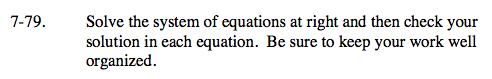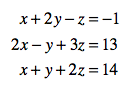Home > A2C > Chapter 7 > Lesson 7.1.5 > Problem7-79

7-79.

Solve the system of equations below and then check your solution in each equation. Be sure to keep your work well organized. Homework Help ✎ x + 2yz = −1
2xy + 3z = 13
x + y + 2z = 14Decide which variable to eliminate first. Use two of the equations to eliminate that variable.

Use the equation you did not use yet and one of the others, and then eliminate the same variable.

Solve the system of two equations in two variables that you created.
Use your results to find the value of the third variable.

(−1, 3, 6)